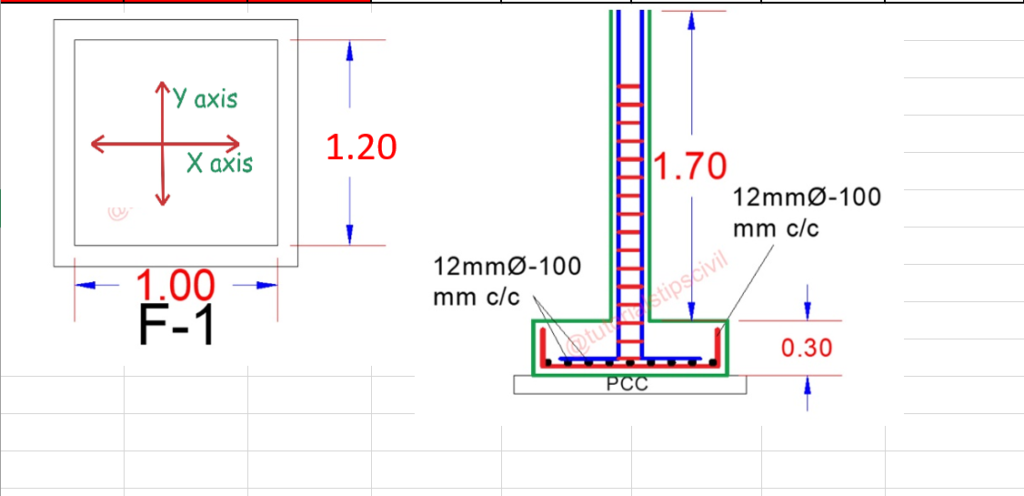# How to calculate BBS of Rectangular Footing with few Easy Step

In this post you can learn simple rectangular footing BBS (Bar Bending Schedule) Just Follow 4 Step one by one.

### How to make BBS on Rectangular Footing.

Calculate BBS of Footing Just 4 Step

Given Data From Footing Drawing

Footing Size  = 1 m x 1.2 m
Spacing of Bar  = 100 mm C/c
Footing Cover  = 50 mm
Bar Use in Footing = 12 mmStep- 1
Cutting Length of Y-Axis

Cutting Length = Length (Y-Axis) – (2x Cover) + (2x Height)  – 4 Cover (For  “L” Upside)  – Bend

Cutting Length = {1200 – (2 x 50) }+ (2 x 300) – (4 x 50) – (2 x 2 x 12)

Cutting Length = 1100 + 600 – 200 – 48

Cutting Length = 1100 + 600 – 248

Cutting Length = 1700 – 248

Cutting Length = 1452

Cutting Length = 1.45 m

Step- 2
Cutting Length of X-Axis

Cutting Length = Length (X-Axis) – (2x Cover) + (2x Height)  – 4 Cover (For  “L” Upside)  – Bend

Cutting Length = {1000-(2 x 50)} + (2 x 300) – (4 x 50) – (2 x 2 x 12)

Cutting Length = 900 + 600 – 200 – 48

Cutting Length = 900 + 600 – 248

Cutting Length = 1500 – 248

Cutting Length= 1252

Cutting Length= 1.25 m

Step- 3
Numbers of bar Along With X Axis ( Length – 1.45 m)

Number of Bar X-Axis = [ Length – (2x Cover) ] /Spacing + 1

Number of Bar X-Axis = [ 1000 – (2x 50) ] /100 + 1

Number of Bar X-Axis = ( 900 / 100 ) + 1

Number of Bar X-Axis = 10 Nos

We Need 10 Number of bar for length of 1.45 m.

Step- 4
Numbers of bar Along With Y Axis ( Length – 1.25 m)

Number of Bar X-Axis = [ Length – (2x Cover) ] /Spacing + 1

Number of Bar X-Axis = [ 1200 – (2x 50) ] /100 + 1

Number of Bar X-Axis = ( 1100 / 100 ) + 1

Number of Bar X-Axis = 12 Nos

We Need 12 Number of bar for length of 1.25 m.You Can Also learn about stirrups Cutting Length.

Visit ourChannel for more information.

Tutorials tips

### 10 Responses

1.ramesh says:

sir what is 2x2x12 i am not able to ubderstand

•Nishad says:

Sir it is = band . for 90degree bend it is 2*d(12mm dia of bar). So we have 2 bends(90degree bends). So it is = 2*2*12

•Tutorial Tips Civil says:

2 no. of bend and 90 degree bend for 2 D and d is dia of bar is 12 mm

2.ramesh says:

sir what is 2x2x12 i am not able to understand please explain

3.Balbir Singh says:

Sir aap rigid road construction ke baare mai bhi bataao

4.Tutorial Tips Civil says:

2 no. of bend and 90 degree bend for 2 D and d is dia of bar is 12 mm

5.Tutorial Tips Civil says:

ache se samjhna hai to please watch my youtube channel waha aapke saaare doubt clear ho jayenge.

6.Henry Acquah says:

I’m really getting the facts

7.Civil Tutorials says:

Sir mujhe isse bahut help mili hai thank you so much and apka YouTube se bhi bahut knowledge mila h

8.RAHUL Kushwaha says:

Thanks sir for sharing